# A chemistry teacher needs to make 2.30 L of a potassium chloride solution for an experimen...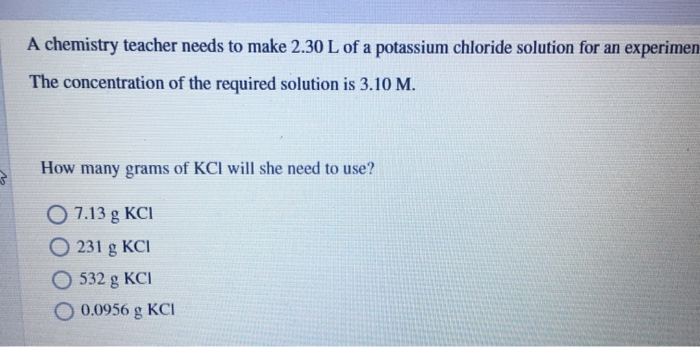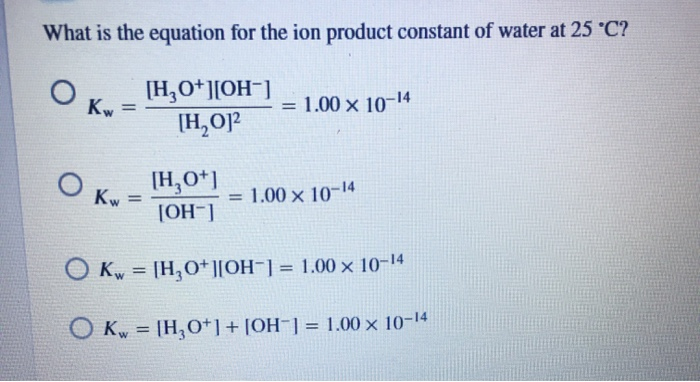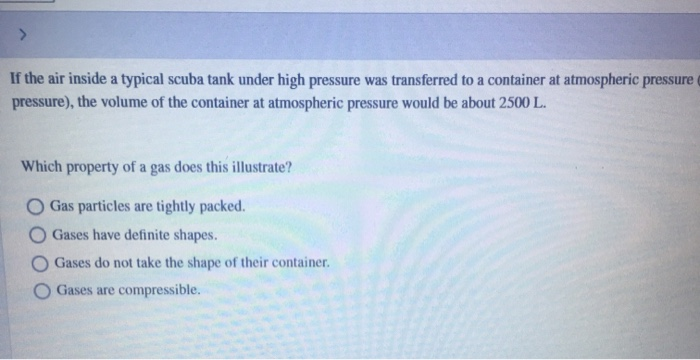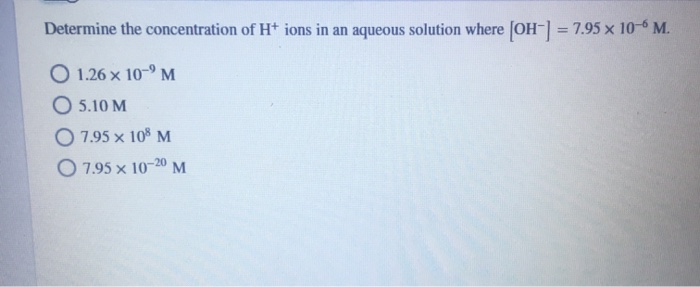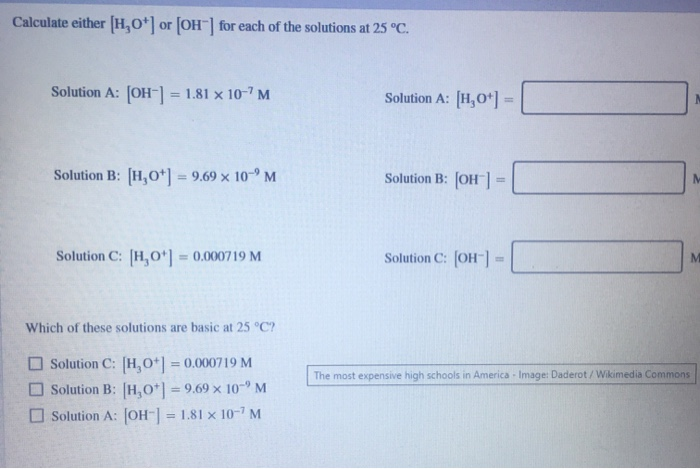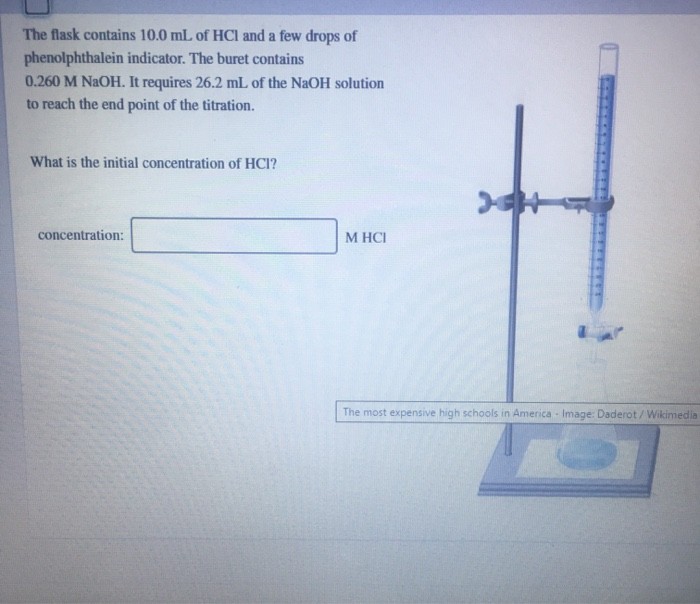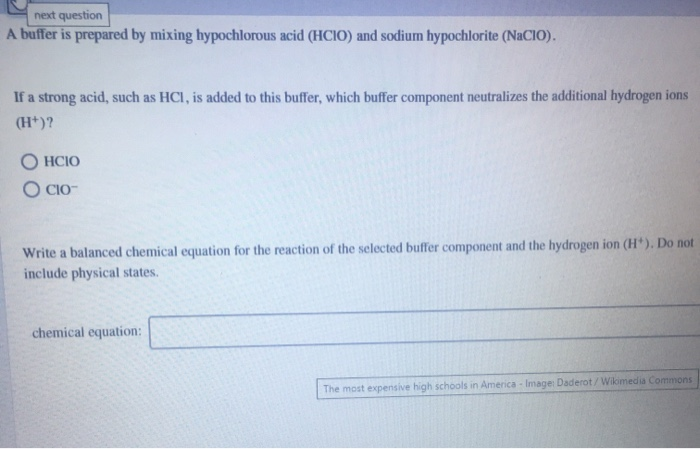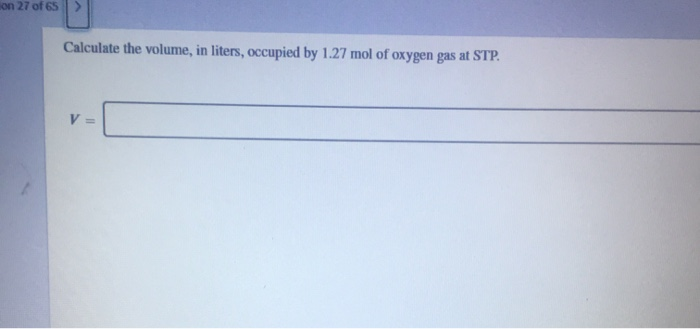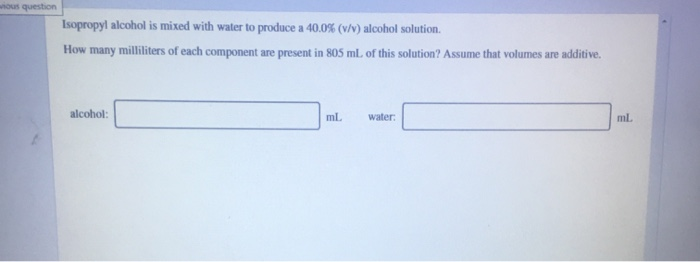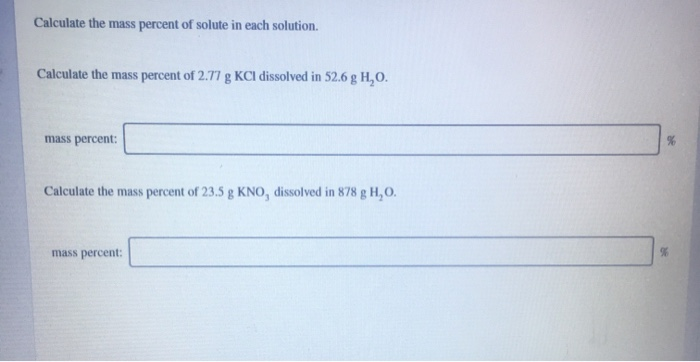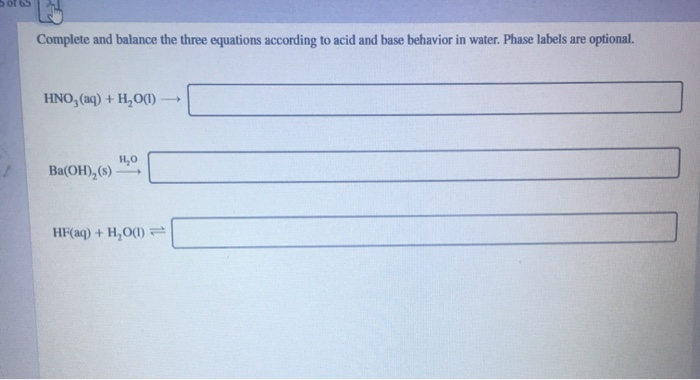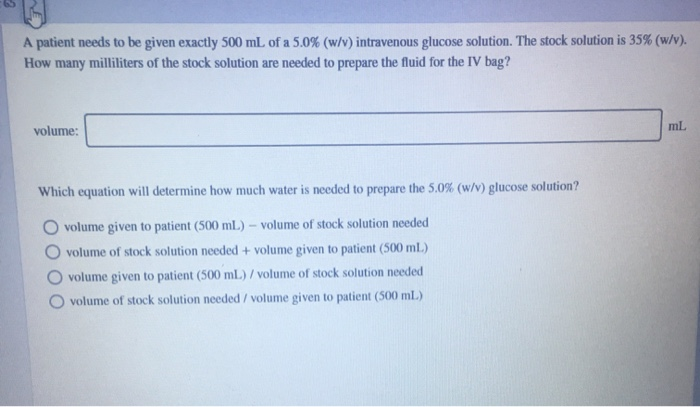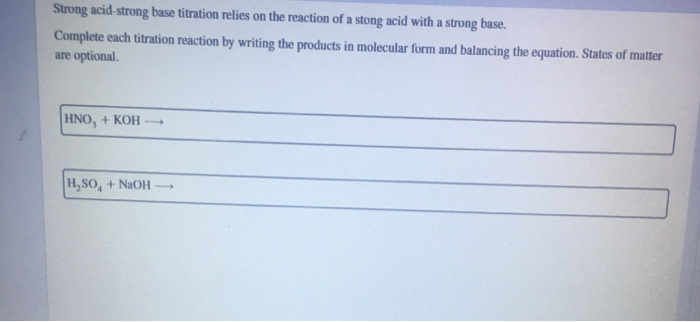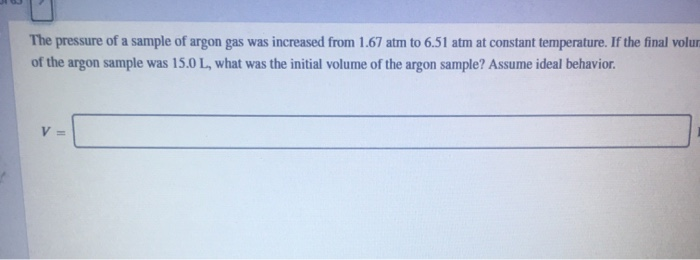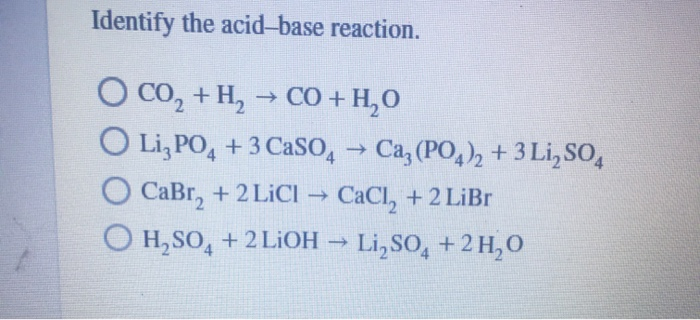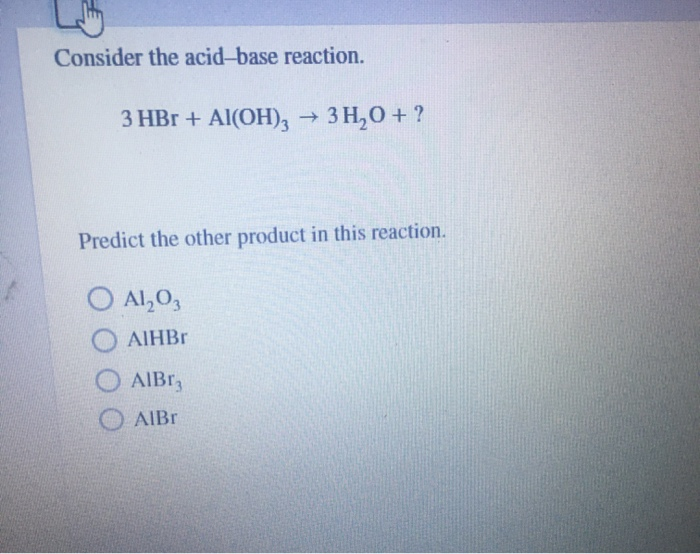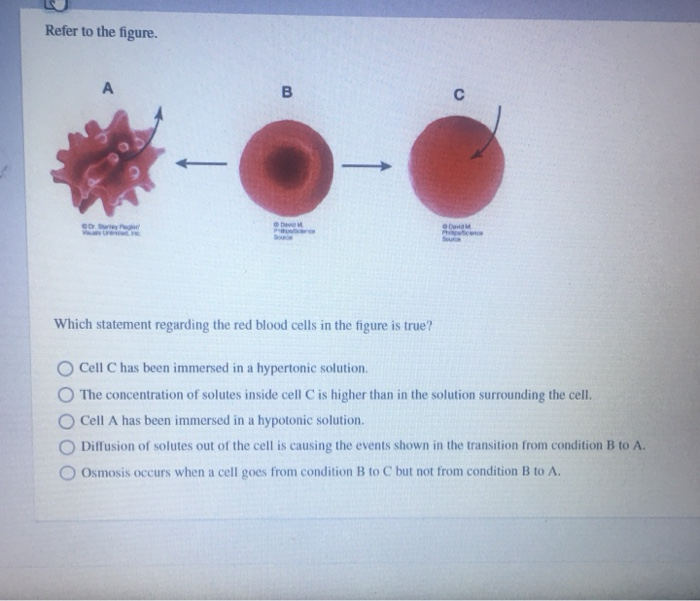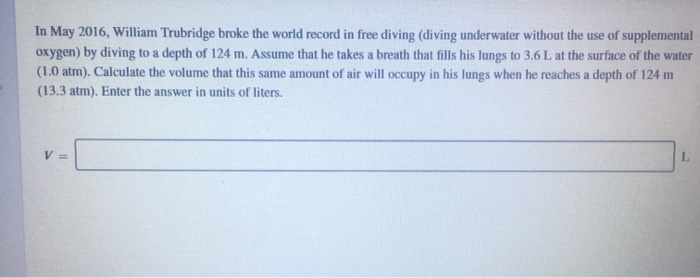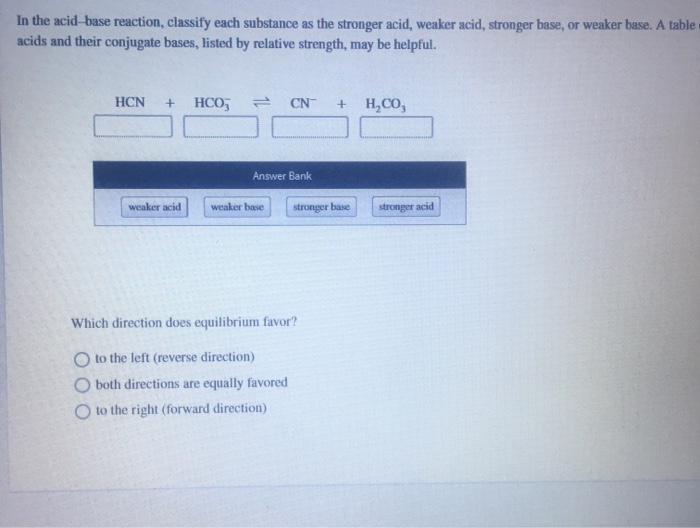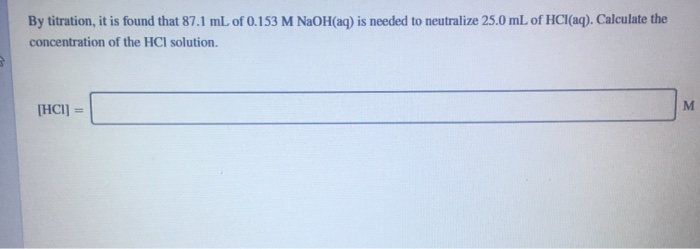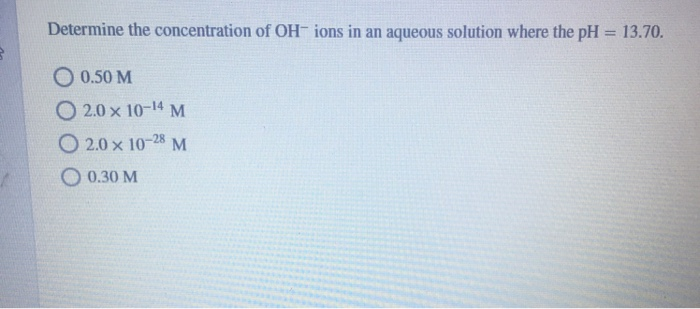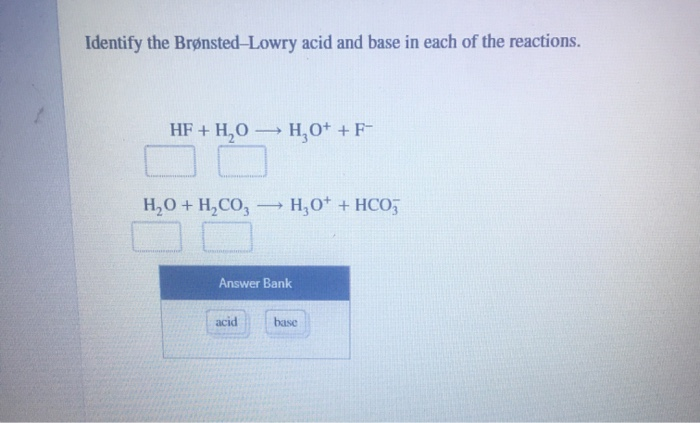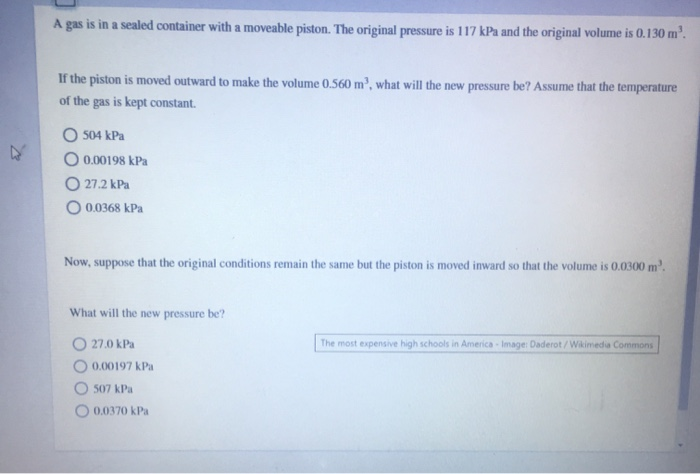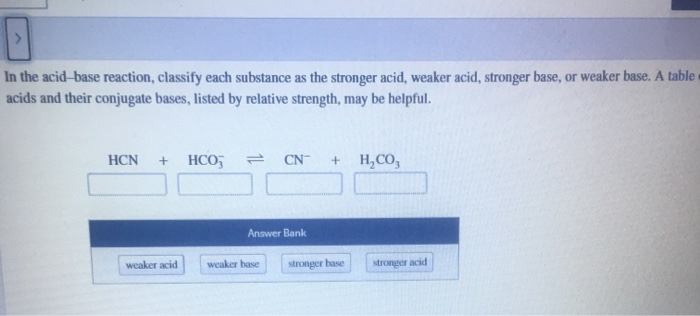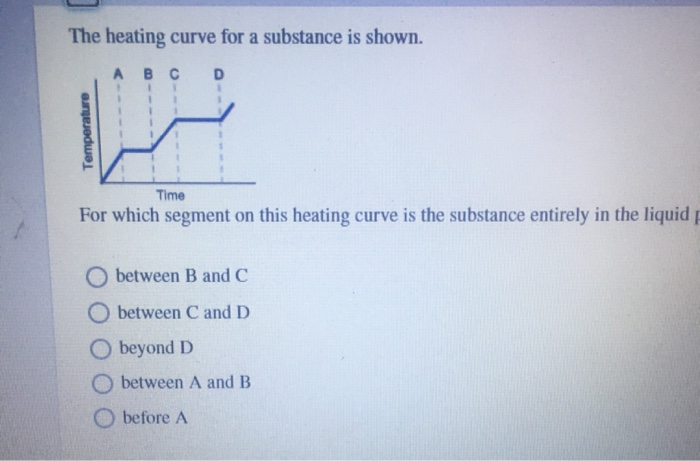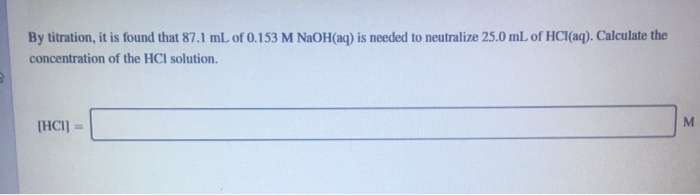A chemistry teacher needs to make 2.30 L of a potassium chloride solution for an experimen The concentration of the required solution is 3.10 M. How many grams of KCI will she need to use? 0 7.13 g KCI 0 231 g KCI O 532 g KCI 0.0956 g KCI
What is the equation for the ion product constant of water at 25 °C? Kw [H,0+][OH-] [H,O] = 1.00 x 10-14 Kw [H,0+1 [OH-] = 1.00 x 10-14 Kw = [H, 0+][OH-] = 1.00 x 10-14 O Kw = [H,0+1+ [OH-] = 1.00 x 10-14
> If the air inside a typical scuba tank under high pressure was transferred to a container at atmospheric pressure pressure), the volume of the container at atmospheric pressure would be about 2500 L. Which property of a gas does this illustrate? Gas particles are tightly packed. O Gases have definite shapes. O Gases do not take the shape of their container. Gases are compressible.
Determine the concentration of H+ ions in an aqueous solution where [OH-] = 7.95 x 10-6 M. O 1.26 x 10-'M 05.10 M O 7.95 x 108 M 7.95 x 10-20 M
Calculate either [H0] or [OH") for each of the solutions at 25 °C. Solution A: [OH-] = 1.81 x 10-?M Solution A: [H,0+1 = Solution B: [H,0*) = 9.69 x 10-M Solution B: (OH) Solution C: [H,0*] = 0.000719 M Solution C: (OH) Which of these solutions are basic at 25°C? The most expensive high schools in America - Image: Daderot / Wikimedia Commons Solution C: [H0+) = 0,000719 M Solution B: H,0*) = 9.69 x 10-"M Solution A: [OH-] = 1.81 x 10-7M
The flask contains 10.0 mL of HCl and a few drops of phenolphthalein indicator. The buret contains 0.260 M NaOH. It requires 26.2 mL of the NaOH solution to reach the end point of the titration. What is the initial concentration of HCI? concentration: МНСІ The most expensive high schools in America - Image: Daderot / Wikimedia
next question A buffer is prepared by mixing hypochlorous acid (HCIO) and sodium hypochlorite (NaCIO). If a strong acid, such as HCl, is added to this buffer, which buffer component neutralizes the additional hydrogen ions (H+)? HCIO OCIO- Write a balanced chemical equation for the reaction of the selected buffer component and the hydrogen ion (H+). Do not include physical states chemical equation: The most expensive high schools in America - Images Daderot / Wikimedia Commons
on 27 of 65 Calculate the volume, in liters, occupied by 1.27 mol of oxygen gas at STP. V
wous question Isopropyl alcohol is mixed with water to produce a 40.0% (v/v) alcohol solution. How many milliliters of each component are present in 805 mL of this solution? Assume that volumes are additive. alcohol: ml water: ml
Calculate the mass percent of solute in each solution. Calculate the mass percent of 2.77 g KCl dissolved in 52.6 g H,O. mass percent Calculate the mass percent of 23.5 g KNO, dissolved in 878 g H,O, mass percent:
Complete and balance the three equations according to acid and base behavior in water. Phase labels are optional. HNO, (aq) + H2O(1) 1,0 Ba(OH),(s) HF(aq) + H2O(1)
A patient needs to be given exactly 500 mL of a 5.0% (w/v) intravenous glucose solution. The stock solution is 35% (w/v). How many milliliters of the stock solution are needed to prepare the fluid for the IV bag? volume: ml Which equation will determine how much water is needed to prepare the 5.0% (w/v) glucose solution? volume given to patient (500 mL) - volume of stock solution needed volume of stock solution needed + volume given to patient (500 ml.) volume given to patient (500 mL) / volume of stock solution needed volume of stock solution needed / volume given to patient (500 ml)
Strong acid-strong base titration relies on the reaction of a stong acid with a strong base. Complete each titration reaction by writing the products in molecular form and balancing the equation. States of matter are optional HNO, + KOH HSO4 + NaOH =
The pressure of a sample of argon gas was increased from 1.67 atm to 6.51 atm at constant temperature. If the final volun of the argon sample was 15.0 L, what was the initial volume of the argon sample? Assume ideal behavior. V=
Identify the acid-base reaction. -> O CO, +H, → CO+H,0 O Li, PO4 + 3 Caso Caz(PO4)2 + 3 Li,SO4 CaBr, + 2 LiCl → CaCl, + 2 LiBr OH,504 + 2 LiOH – Li,SO4 + 2 H, 0
Consider the acid-base reaction. 3 HBr + Al(OH)2 + 3H,0+? Predict the other product in this reaction. O Al₂O₃ AIHBr AIBr, AIBI
Refer to the figure. A B С ODS OM Which statement regarding the red blood cells in the figure is true? Cell C has been immersed in a hypertonic solution. The concentration of solutes inside cell C is higher than in the solution surrounding the cell. Cell A has been immersed in a hypotonic solution. Diffusion of solutes out of the cell is causing the events shown in the transition from condition B to A. Osmosis occurs when a cell goes from condition B to C but not from condition B to A.
In May 2016, William Trubridge broke the world record in free diving (diving underwater without the use of supplemental oxygen) by diving to a depth of 124 m. Assume that he takes a breath that fills his lungs to 3.6L at the surface of the water (1.0 atm). Calculate the volume that this same amount of air will occupy in his lungs when he reaches a depth of 124 m (13.3 atm). Enter the answer in units of liters. V=
In the acid-base reaction, classify each substance as the stronger acid, weaker acid, stronger base, or weaker base. A table acids and their conjugate bases, listed by relative strength, may be helpful. HCN + HCO; CN- + H.CO Answer Bank weaker acid weaker base stronger base stronger acid Which direction does equilibrium favor? to the left (reverse direction) both directions are equally favored to the right (forward direction)
By titration, it is found that 87.1 mL of 0.153 M NaOH(aq) is needed to neutralize 25.0 mL of HCl(aq). Calculate the concentration of the HCl solution. M [HCI)
Determine the concentration of OH-ions in an aqueous solution where the pH = 13.70. O 0.50 M 0 2.0 x 10-14 M O 2.0 x 10-28 M 0.30 M
Identify the Brønsted-Lowry acid and base in each of the reactions. HF + H2O H0+ +F- H,0 + H,CO, — H, 0+ + HCO3 Answer Bank acid base
A gas is in a sealed container with a moveable piston. The original pressure is 117 kPa and the original volume is 0.130 m'. If the piston is moved outward to make the volume 0.560 m', what will the new pressure be? Assume that the temperature of the gas is kept constant O 504 kPa O 0.00198 kPa 27.2 kPa O 0.0368 kPa Now, suppose that the original conditions remain the same but the piston is moved inward so that the volume is 0.0300 m² The most expensive high schools in America - Image: Daderot/Wikimedia Commons What will the new pressure be? 27.0 kPa 0.00197 kPa 507 kPa 0.0370 kPa
In the acid-base reaction, classify each substance as the stronger acid, weaker acid, stronger base, or weaker base. A table acids and their conjugate bases, listed by relative strength, may be helpful. HCN + HCO3 CN + H,CO, Answer Bank weaker acid weaker base stronger base stronger acid
The heating curve for a substance is shown. A B C D 1 1 Temperature Time For which segment on this heating curve is the substance entirely in the liquid between B and C between C and D Obeyond D between A and B before A
By titration, it is found that 87.1 mL of 0.153 M NaOH(aq) is needed to neutralize 25.0 mL of HCl(aq). Calculate the concentration of the HCI solution. [HCI) M

1. Given data:

M = 3.10 M

V = 2.30 L

Molar mass of KCl = (39 + 35.5 ) g/mol = 74.5 g/mol

Molarity (M) = no. of moles of solute (n) / volume of solution V in litre ------- (1)

no. of moles (n) = given mass / Molar mass --------(2)

Using equation (2) in equation (1)

M = (given mass / molar mass ) / V

3.10 = (given mass / 74.5) / 2.30

given mass = 531.18 g of KCl

Correct option is third (532 g).

2. H2O (l) + H2O (l)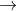H3O+ (aq) + OH- (aq)

K = [H3O+ (aq)] [OH- (aq)] / [H2O (l)] [H2​​​​​​​O (l)]

Concentration of water is taken as 1 as it is in pure liquid form and in huge amount.

Kw = [H3O+ (aq)] [OH- (aq)] = 1.00 x 10-14

Correct option is third.

3. Gases are easily compressible as molecules have great distances between each other and due to which they can be stored under high pressure and a small container can contain large volume of gas.

Correct option is fourth (gases are compressible).

4. Kw = [H+] [OH-] = 10-14

[H+] = 10-14 / [OH-]

= 10-14 / 7.95 x 10-6

= 0.126 x 10-8 = 1.26 x 10-9 M

Correct option is first (1.26 x 10-9 M) .

#### Earn Coin

Coins can be redeemed for fabulous gifts.

Similar Homework Help Questions
• ### What is the pH n aqueous solution with a hydroxide ion concentration of 1.60 x 10-4...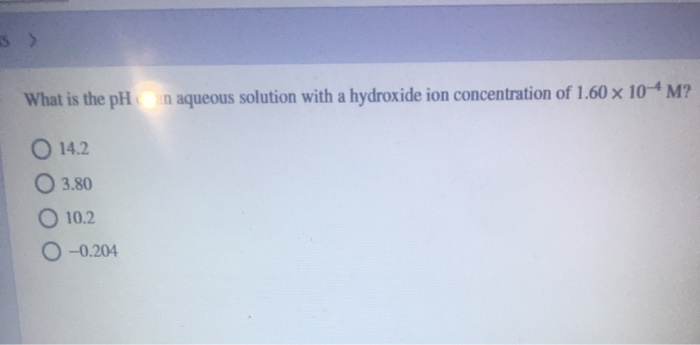What is the pH n aqueous solution with a hydroxide ion concentration of 1.60 x 10-4 M? O 14.2 03.80 O 10.2 0 -0.204 Consider the reaction. HF(aq) + KOH(aq) — KF(aq) + H20(1) What is the net ionic equation for the chemical reaction? HF(aq) + K+ (aq) + OH(aq) — K+(aq) + F-(aq) + H+(1) + OH-(1 HF(aq) + K+ (aq) —> K+ (aq) + H2O(l) HF(aq) + K+(aq) + OH- (aq) — K+(aq) +F-(aq) + H2O(1) HF(aq) +...

• ### A chemistry teacher needs to make 2.30 L of a potassium chloride solution for an experiment....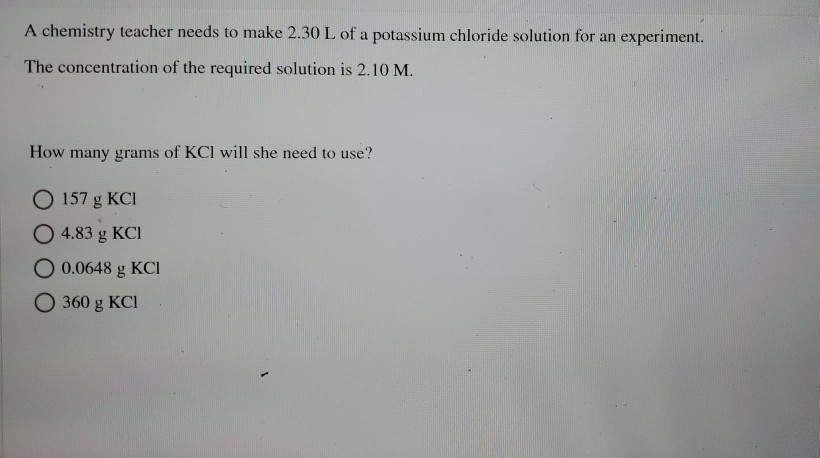A chemistry teacher needs to make 2.30 L of a potassium chloride solution for an experiment. The concentration of the required solution is 2.10 M. How many grams of KCI will she need to use? O 157 g KCI O 4.83 g KCI O 0.0648 g KCI O 360 g KCI

• ### By titration, it is found that 87.1 mL of 0.153 M NaOH(aq) is needed to neutralize...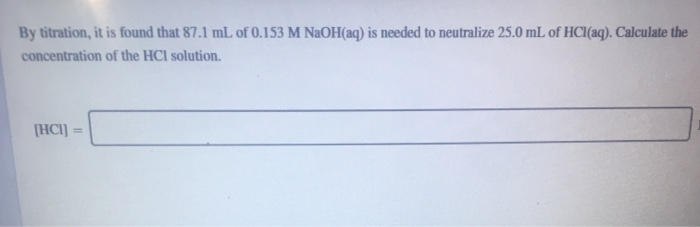By titration, it is found that 87.1 mL of 0.153 M NaOH(aq) is needed to neutralize 25.0 mL of HCl(aq). Calculate the concentration of the HCl solution. (HCI) In the acid reaction, classify each substance as the stronger acid, weaker acid, stronger base, or weaker base. A table of acids and their conjugate bases, listed by relative strength, may be helpful. HCN + HCO3 CN + H,CO Answer Bank weaker acid stronger base stronger acid weaker base Which direction does...

• ### of 65 > A sample of helium at 20°C occupies a volume of 6.09 L at...of 65 > A sample of helium at 20°C occupies a volume of 6.09 L at a pressure of 8.00 bar. What volume does this helium sample occupy if the pressure is reduced to 7.36 bar while maintaining the temperature at 20°C? volume: L The most expensive high schools in America - Image: Daderot/Wikimedia Comm about privacy policy hes contact us An aerosol can is empty, except for the propellant gas which exerts a pressure of 1.20 atm at 22...

• ### Experiment 13 - Acid and Bases Worksheet 1. Arrhenius theory acid-base reactions can also be classified...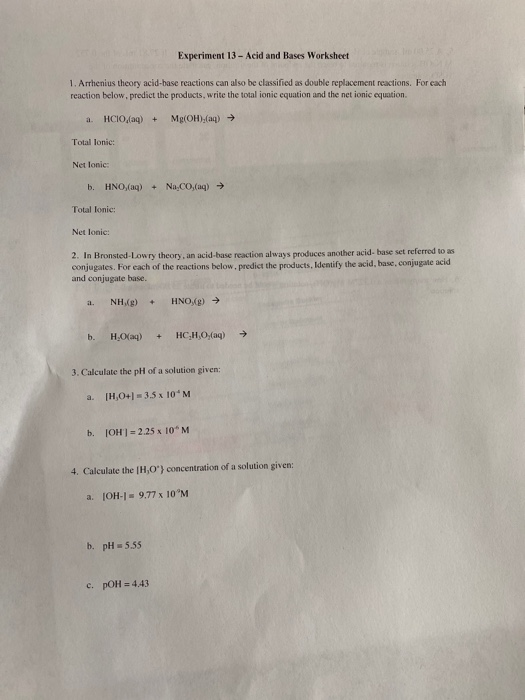Experiment 13 - Acid and Bases Worksheet 1. Arrhenius theory acid-base reactions can also be classified as double replacement reactions. For each reaction below. predict the products, write the total ionic equation and the net ionic equation HCIO (aq) + Mg(OH),(aq) Total lonic: Net lonic: b. HNO,(aq) + Na CO (aq) → Total lonie: Net lonie: 2. In Bronsted-Lowry theory, an acid-base reaction always produces another acid base set referred to as conjugales. For each of the reactions below. predict...

• ### 1-Each of the following metals reacts with nitric acid to form the metal chloride and hydrogen gas. Complete and bal- an...

1-Each of the following metals reacts with nitric acid to form the metal chloride and hydrogen gas. Complete and bal- ance the reactions to show the formation of these products. a. Zn (s) +HNO, (aq)-> b. Pb (s) +HNO3 (aq) - c. Na (s) HNO3 (aq)- d. Al (s) HNO, (aq)- 2- Complete and balance these acid-base neutralization reactions: a. CSOH (aq) HBr (aq) b. HBr (aq) Fe(OH)2 (s) c. H,PO4 (aq) +KOH (aq)- 3-In a titration experiment, two drops...

• ### 12. Given that Ka = 1.8 x 10- for acetic acid. in order for an acetic...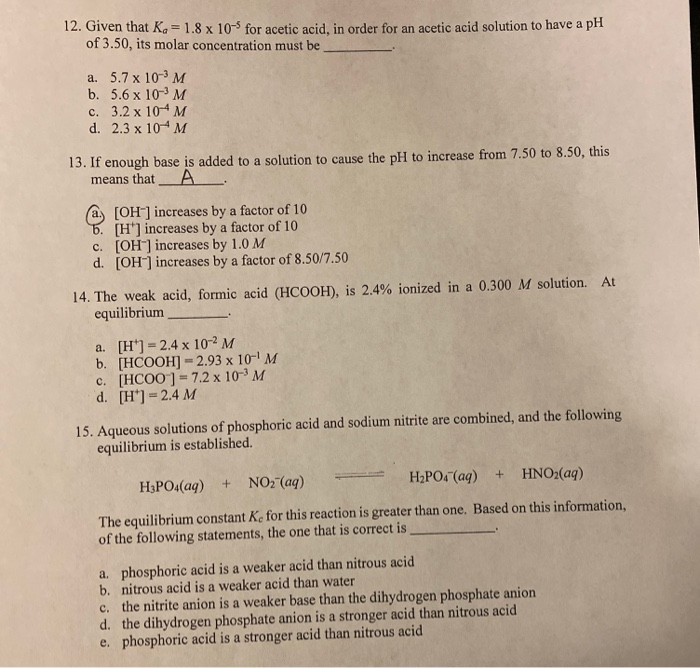12. Given that Ka = 1.8 x 10- for acetic acid. in order for an acetic acid solution to have a pH of 3.50, its molar concentration must be a. 5.7 x 10-3 M b. 5.6 x 10-3M c. 3.2 x 10-4M d. 2.3 x 10-M 13. If enough base is added to a solution to cause the pH to increase from 7.50 to 8.50, this means that А a. [OH-] increases by a factor of 10 b. [H'] increases...

• ### Ca(OH)2 (aq) + 2HCl (aq) CaCl2 (aq) + H2​O (l) An aqueous solution of Ca(OH)2with a...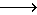Ca(OH)2 (aq) + 2HCl (aq) CaCl2 (aq) + H2​O (l) An aqueous solution of Ca(OH)2with a concentration of 0.161 M was used to titrate 25.00 mL of aqueous HCl. 18.63 mL of the Ca(OH)2was required to reach the endpoint of the titration. An aqueous solution of Ca(OH)2with a concentration of 0.161 M was used to titrate 25.00 mL of aqueous HCl. 18.63 mL of the Ca(OH)2was required to reach the endpoint of the titration. A) How many moles of base...

• ### A chemist needs to determine the concentration of a solution of nitric acid, HNO3. She puts...

A chemist needs to determine the concentration of a solution of nitric acid, HNO3. She puts 875 mLof the acid in a flask along with a few drops of indicator. She then slowly adds 0.200 MBa(OH)2 to the flask until the solution turns pink, indicating the equivalence point of the titration. She notes that 145 mL of Ba(OH)2 was needed to reach the equivalence point. Solution map In this titration, the concentration of base is known and can be used...

• ### 5. A 3.5 L sample of a 5.8 M NaCl solution is diluted to 55L. What...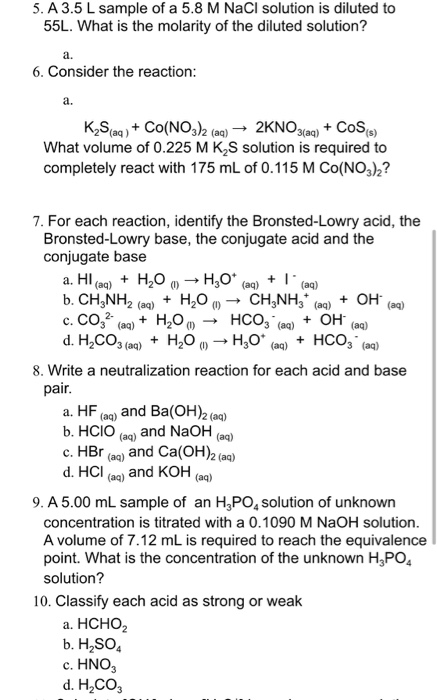5. A 3.5 L sample of a 5.8 M NaCl solution is diluted to 55L. What is the molarity of the diluted solution? 6. Consider the reaction: K Slaq) + Co(NO3)2 (aq) → 2KNO3(aq) + Cos) What volume of 0.225 M K S solution is required to completely react with 175 mL of 0.115 M CO(NO3)2? 7. For each reaction, identify the Bronsted-Lowry acid, the Bronsted-Lowry base, the conjugate acid and the conjugate base a. Hl(aq) + H2O → H30*...

Free Homework App